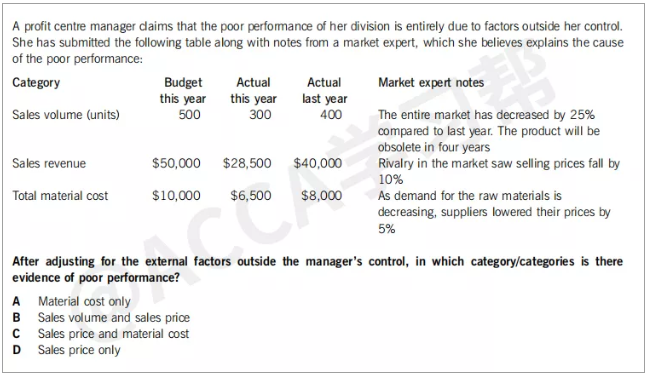PM（F5）科目中關于Variance的兩道真題，你都能做對嗎？

Sept 2016 No.131）Sales volume

Actual：300

Revised：看調整項，比去年下降25%，也就是400*（1-25%）=300，Actual=revised，不算好也不算壞。

2）Sales revenue

Actual:\$28,500

Revised revenue=revised sales volume*revised sales price

Original Budget的單價是\$50,000/500=\$100

100*(1-10%)=\$90

Revised revenue=\$27000&lt;actual revenue=\$28500

3）material cost

PM科目中當題目沒有刻意的說銷量和產量之間差異的時候，都默認產量=銷量

Actual material cost=\$6500

Revised material cost=revised volume*revised material cost per unit

Revised volume=300

revised material cost per unit根據note，下降5%。

Original budget material cost per unit=\$10,000/500=\$20

revised material cost per unit=\$20*(1-5%)=\$19

Revised material cost=300*19=\$5700

Revised material cost=\$5700&gt;actual material cost=\$6500

Specimen 2016 No.5